# Prove that the relation of congruence is an equivalence relation.Question
CongruenceProve that the relation of congruence is an equivalence relation.2020-11-09
Step 1
The definition of a congruence depends on the type of algebraic structure under consideration.
So, for the question “Prove that the relation of congruence is an equivalence relation.” we are taking in general.
Let $$a \equiv b$$ is denoted as a is congruent to b.
Step 2
Now,
Reflexive:
$$Since\ a\ \equiv a\forall a,\ the\ relation\ \equiv is\ reflexive$$.
Symmetry:
$$Since\ a \equiv b\ then\ b \equiv\ a, the\ relation\ \equiv\ is\ symmetry$$.
Transitivity:
$$Since\ a \equiv b\ and\ b \equiv c\ then\ a \equiv c\ ,\ the\ relation\ \equiv is\ transitive$$.
Hence, the relation of congruence is an equivalence relation.

### Relevant QuestionsProve the transitivity of modular congruence. That is, prove that for all integers a,b,c, and n with n > 1, if $$a = b(mod\ n)\ and\ b = c(mod\ n)\ then\ a = c(mod\ n)$$.decide whether enough information is given to prove that the triangles are congruent using the SAS Congruence Theorem.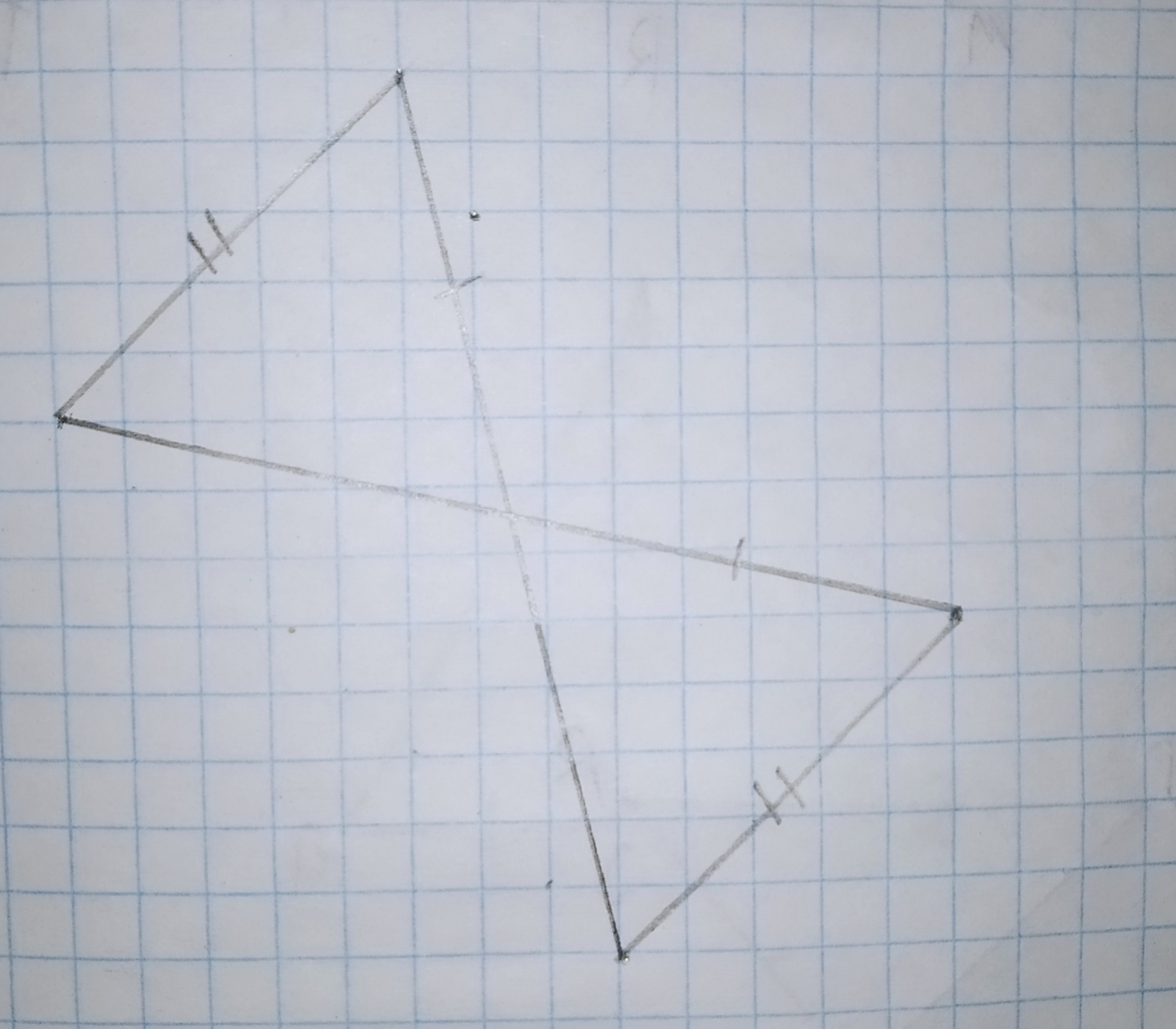Decide whether enough information is given to prove that the triangles are congruent using the SAS Congruence Theorem.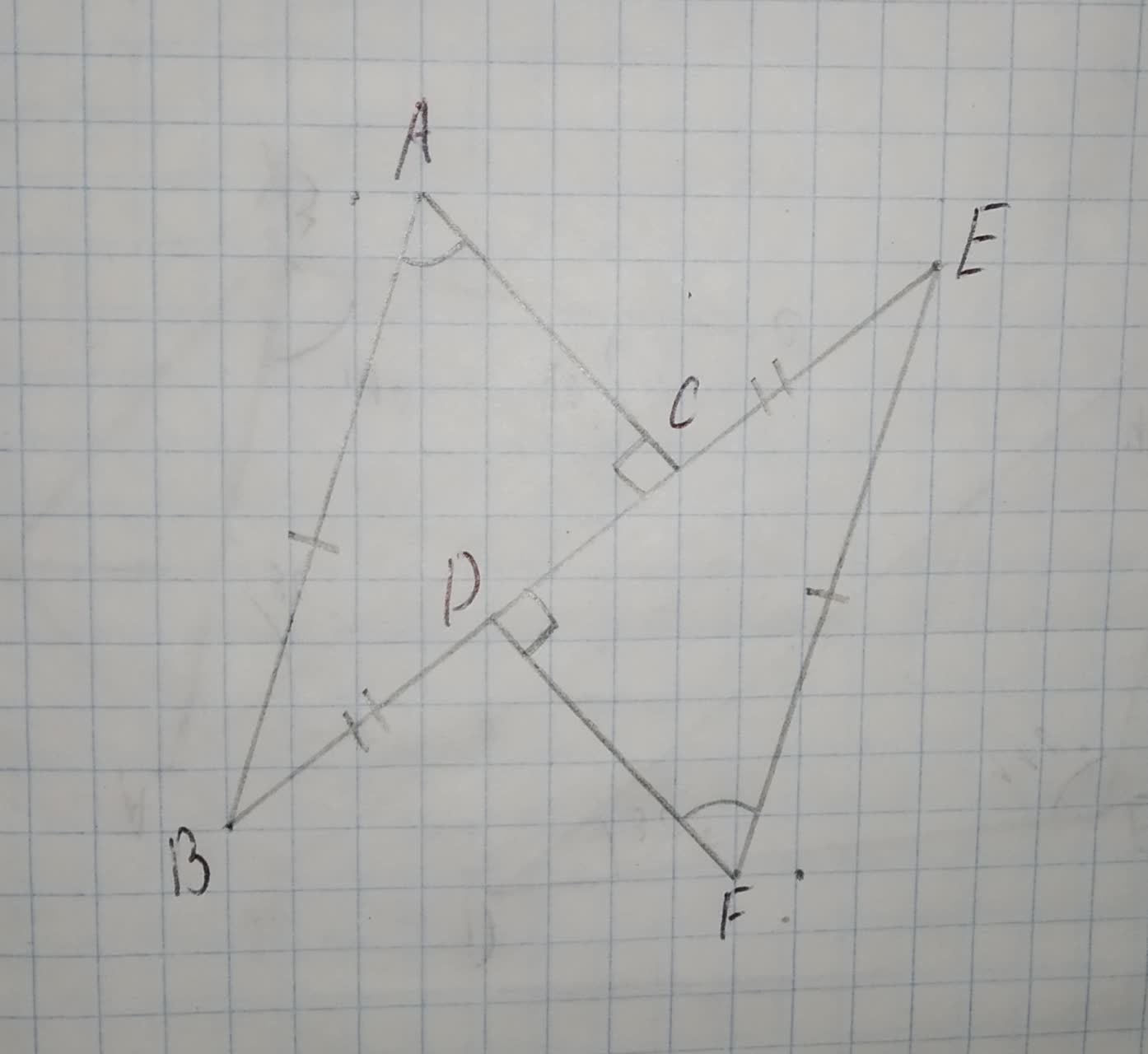Prove directly from the definition of congruence modulo n that if a,c, and n are integers,n >1, and $$\displaystyle{a}\equiv{c}{\left(\text{mod}{n}\right)},{t}{h}{e}{n}{a}^{{3}}\equiv{c}^{{3}}{\left(\text{mod}{n}\right)}$$.Prove that the similarity of polygons is an equivalence relation.State the third congruence required to prove the congruence of triangles using the indicated postulate.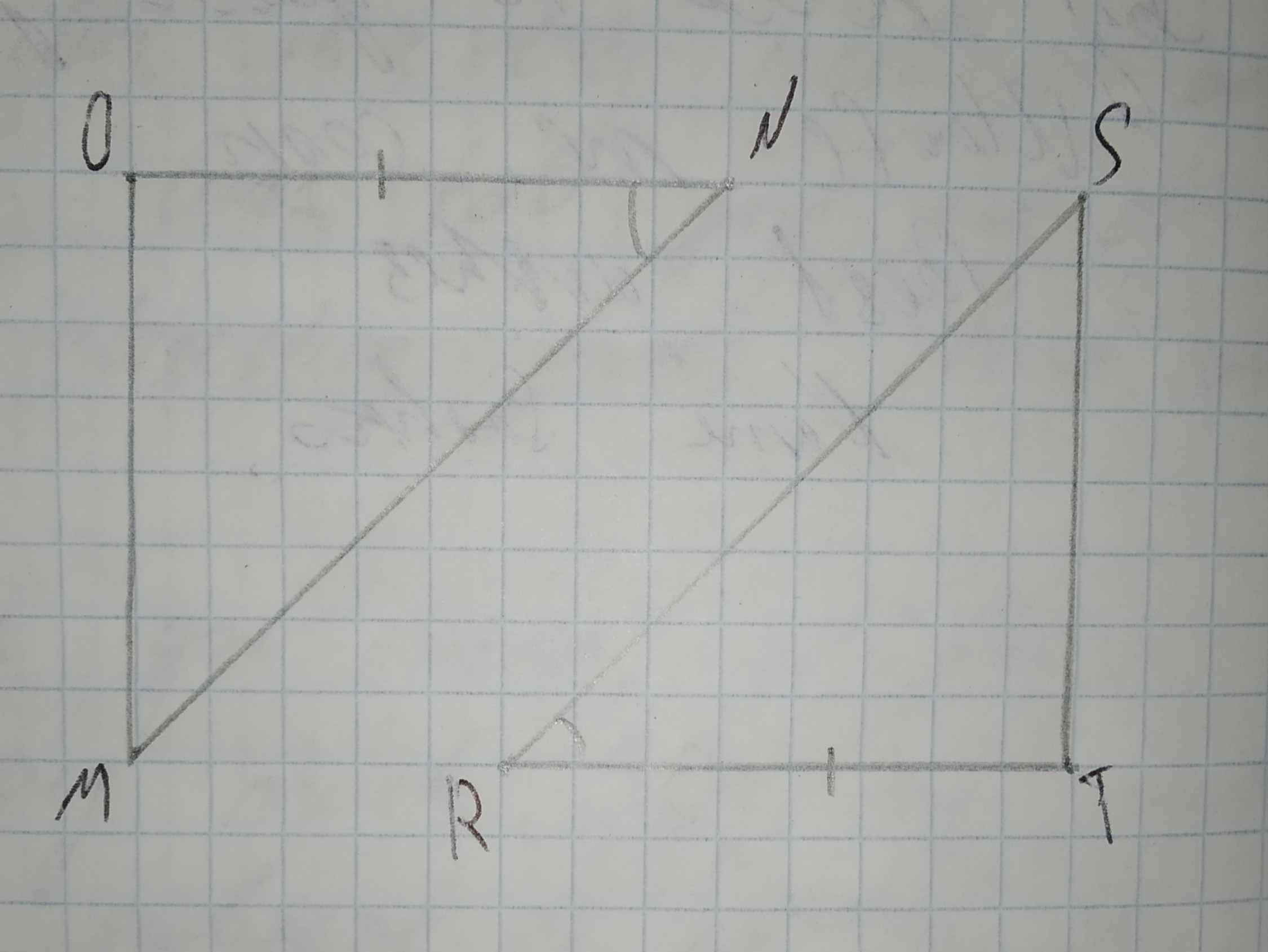a)$$\displaystyle\overline{{{O}{M}}}\stackrel{\sim}{=}\overline{{{T}{S}}}$$
b)$$\displaystyle\angle{M}\stackrel{\sim}{=}\angle{S}$$
c)$$\displaystyle\overline{{{O}{N}}}\stackrel{\sim}{=}\overline{{{T}{R}}}$$
d)$$\displaystyle\angle{O}\stackrel{\sim}{=}\angle{T}$$State the third congruence required to prove the congruence of triangles using the indicated postulate.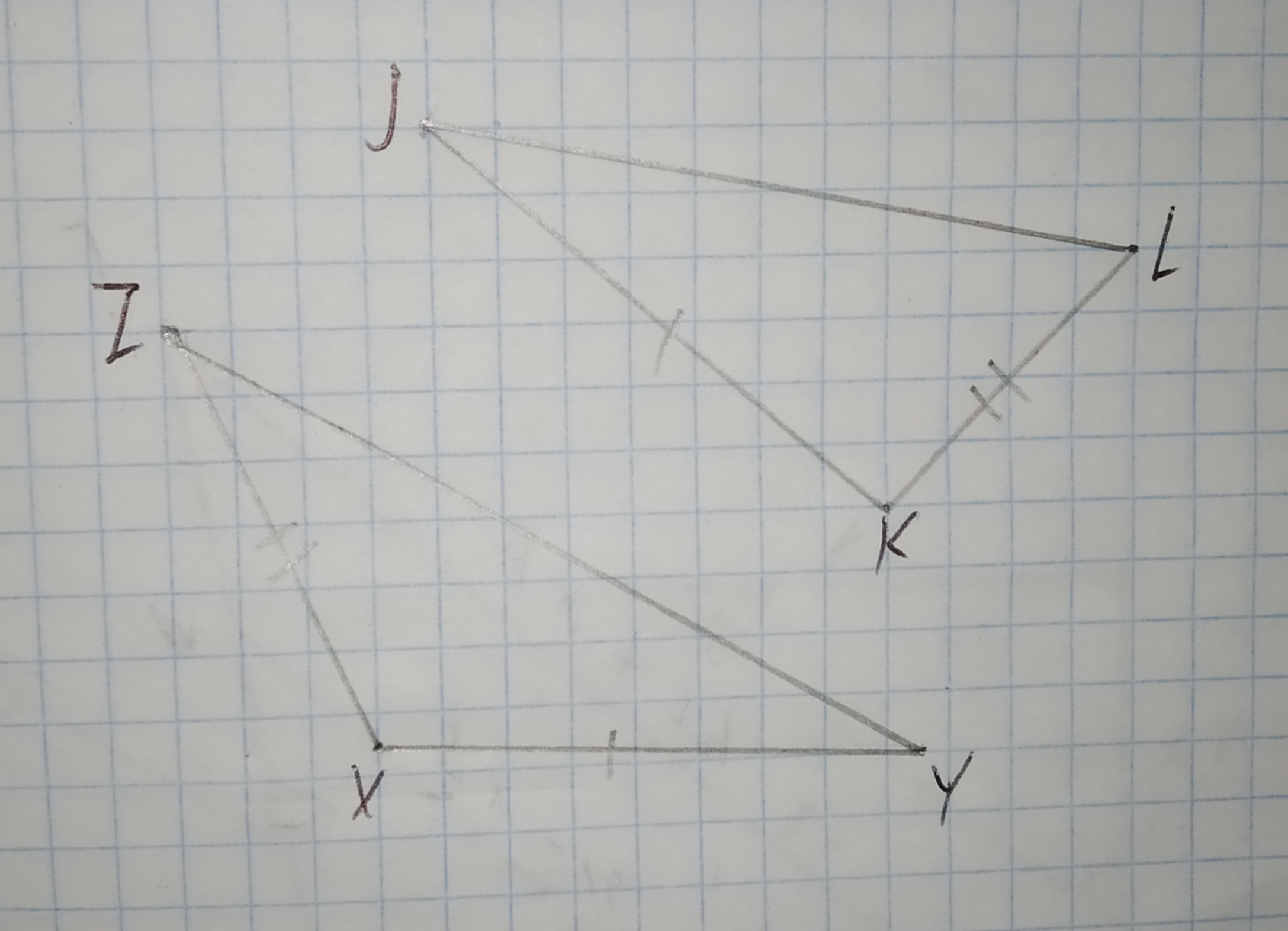a)$$\displaystyle\overline{{{Z}{Y}}}\stackrel{\sim}{=}\overline{{{J}{L}}}$$
b)$$\displaystyle\angle{X}\stackrel{\sim}{=}\angle{K}$$
c)$$\displaystyle\overline{{{K}{L}}}\stackrel{\sim}{=}\overline{{{X}{Z}}}$$
d)$$\displaystyle\angle{Y}\stackrel{\sim}{=}\angle{L}$$With of the following triangle congruence shortcuts could be used to prove PRQ = TRS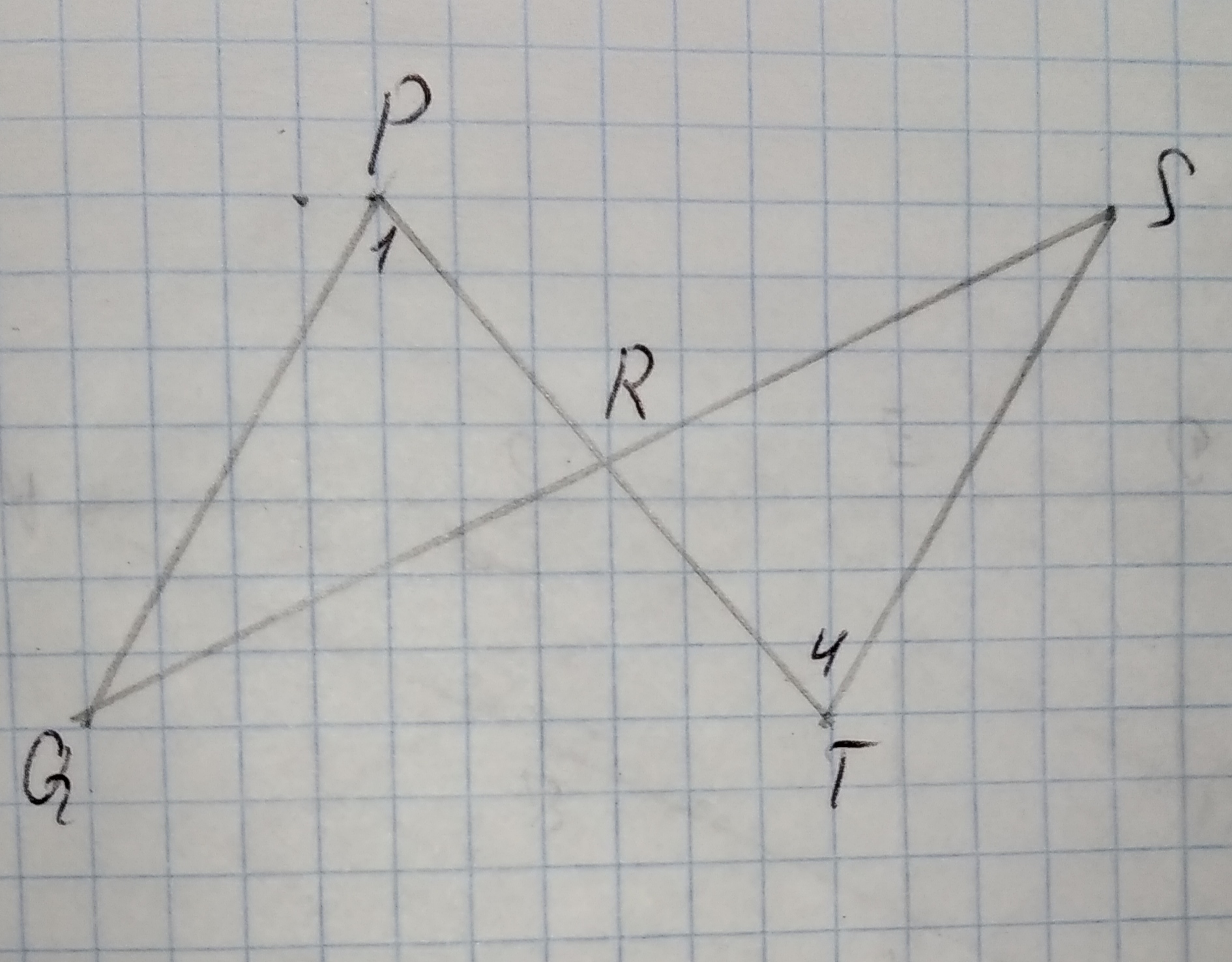Given Data,
$$\displaystyle\angle{Q}\stackrel{\sim}{=}\angle{S}$$
$$\displaystyle\overline{{{Q}{R}}}\stackrel{\sim}{=}\overline{{{S}{R}}}$$
a)Side-Side-Side Postulate (SSS)
b)Side-Angle-Side Postulate (SAS)
c)Angle-Side-Angle Postulate (ASA)
d)Angle-Angle-Side Theorem (AAS)A)$$\displaystyle\angle{B}{A}{C}\stackrel{\sim}{=}\angle{D}{A}{C}$$
B)$$\displaystyle\overline{{{A}{C}}}\stackrel{\sim}{=}\overline{{{B}{D}}}$$
C)$$\displaystyle\angle{B}{C}{A}\stackrel{\sim}{=}\angle{D}{C}{A}$$
D)$$\displaystyle\overline{{{A}{C}}}\stackrel{\sim}{=}\overline{{{B}{D}}}$$